# How to Show/Keep Only Duplicate Values in Excel

Suppose we have one list of some fruits, and some of them are duplicate. If we want to only keep duplicate fruits and remove the unique ones, how can we do? This article will introduce you two methods to only keep duplicate values from a list in excel. The first method, you can use Conditional Formatting function in excel; the second one, you can use formulas with Filter function to keep duplicate values.

Precondition:

Prepare a list A. It contains some duplicate values. We want to keep these duplicate values and remove the others.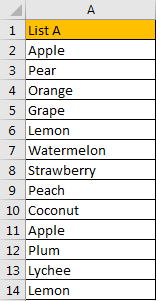## Method 1: Keep Duplicate Values by Conditional Formatting

Step 1: Select List A.

Step 2: Click Home in ribbon, click Conditional Formatting in Styles group.Step 3: In Conditional Formatting dropdown list, select Highlight Cells Rules->Duplicate Values.Step 4: In Duplicate Values dialog, select Duplicate in dropdown list. Keep default value in values with dropdown list. Then click OK.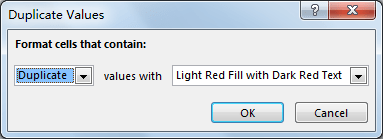Step 5: Verify that all duplicate values are marked with dark red properly.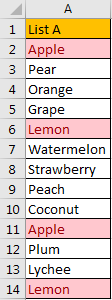Step 6: Select A1, click Data in ribbon, then click Filter in Sort & Filter group.Step 7: Click on filter arrow on A1 to load filter settings. Click Filter by Color, under Filter by Cell Color select cell with color.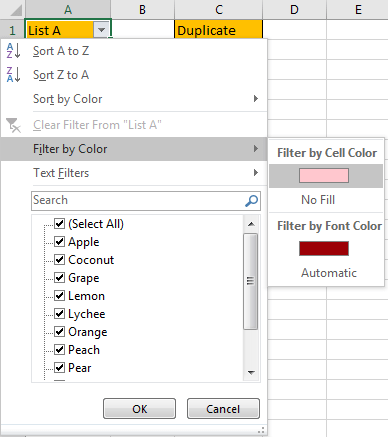Verify that all duplicate values are filtered.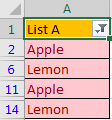Step 8: Now we just need to copy these values to new column. Select these cells, press F5, click Special on Go To dialog.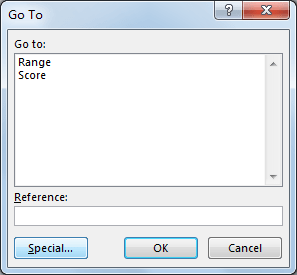Check on Visible cells only, then click OK.Verify that duplicate values are auto activated after above step.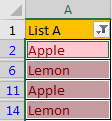Copy and paste them in column B. Clear filter to expand the list. Delete column A. Then only duplicate values are kept.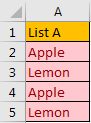## Method 2: Extract Duplicate Values by Formulas

Step 1: In B2 enter the formula =COUNTIFS(\$A\$2:\$A\$14,\$A2)>1. We get TRUE.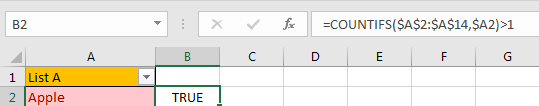Step 2: Drag the fill handle down. Verify that B2:B14 are filled with TRUE or FALSE.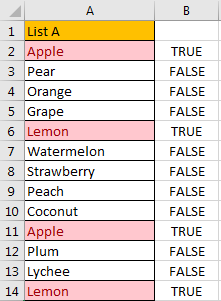Step 3: In B1, click Data tab in ribbon, and click Filter to create a filter. In filter, only check on TRUE, then click OK.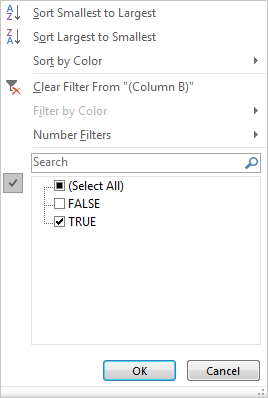Step 4: Verify that only duplicate values are shown.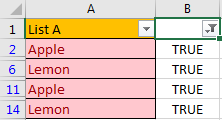Step 5: Repeat step#8 in method 1. Then only duplicate values are kept.

Related Posts

Cap percentage values between 0 and 100

This article will talk about how to cap the percentage values between 0% and 100% in Microsoft Excel Spreadsheet or Google Sheets. If you are a newbie on Excel or google Sheets, you may be able to do this by ...

Calculate Cap Percentages to Specific Value

This article will talk about how to limit the cap percentage of a given amount to a given value in Microsoft Excel Spreadsheet or Google Sheets. If you are a newbie on Excel or google Sheets, you may be able ...

Calculate Win Loss Tie

Suppose you got a task to calculate the win, loss, and tie totals; what would you do? If you are new to Ms Excel and don't have enough experience with it, then you might do this task manually but let ...

Calculate Years Between Dates In Ms Excel

If you are an avid Ms Excel user, then you might have come across a task in which you needed to calculate the years between the dates; you might take it easy and do this task manually, which is also ...

Calculate Number of Hours between Two Times

Calculating the difference between two times might be a valuable statistic for subsequent computations or averages, whether you're producing a time sheet for staff or recording personal exercises. While Excel has a plethora of complex functions, including date and time ...

Calculate Loan Interest in Given Year

When you borrow money, you are supposed to repay it gradually. Lenders, on the other hand, want to be compensated for their services and the risk they incur by lending you money. That is, you will not just repay the ...

Calculate Interest Rate for Loan

The interest rate is the fee charged by a lender to a borrower and is expressed as a percentage of the principal—the lent amount. The interest rate on a loan is often expressed as an annual percentage rate, abbreviated as ...

Calculate Interest for Given Period

Using the IPMT function in Excel, we can compute the interest payment on any loan. This step-by-step tutorial will guide Excel users of all skill levels through the process to calculate interest for given period. Finally, the formula: =IPMT(B3/12,1,B5,-B2) The ...

How To Use Excel GCD Function

This post will guide you how to use Excel GCD function with syntax and examples in Microsoft excel. Description The Excel GCD function Returns the greatest common divisor of two or more integers. So you can use the GCD function ...

Calculate A Ratio From Two Numbers In Excel

In elementary mathematics, a ratio is a connection or comparison between two or more integers. For example, ratios are often expressed as ":" to demonstrate the relationship between two numbers. You would think that manually calculating a ratio from two ...

Sidebar